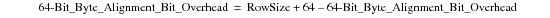# Procedure To Determine the Exact Row Size for Aligned Row Systems - Teradata Database

Product
Release Number
15.10
Language
English (United States)
Last Update
2018-10-06
Product Category
Software

### Procedure To Determine the Exact Row Size for Aligned Row Systems

The following procedure determines the exact row size for aligned row format systems.

1 Set the initial value for RowSize to 12 bytes and the initial value for 64‑Bit_Byte_Alignment_Bit_Overhead to 0 bytes.

2 Record the number of columns compressed on a non-null value.

3 Record the number of nullable columns.

4 Divide the sum of step 2 and step 3 by 8 and record the quotient of the operation.

Call this variable PB.

The purpose of this step is to account for any required additional presence bits that might be required.

For example, if the ratio is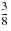, then the quotient is 0.

5 Overwrite the value for RowSize as follows:6 Record the number of variable length columns.

Call this variable VC.

7 Determine the space required for the variable length columns offset array using the following pseudocode procedure:

`     IF VC > 0`
`       THEN`
`         IF (RowSize is odd)`
`           THEN`
`             RowSize += 1`
`             64BitOverhead += 1`
`         ENDIF`
`       RowSize = RowSize + 2 * (VC + 1)`
`     END IF`

where += is the assignment operator.

8 Determine the maximum alignment required for all fixed length columns.

Call this variable FA.

9 Determine the size of all fixed length columns.

Call this variable FS.

10 Determine the maximum alignment required for all compressible columns.

Call this variable CA.

11 Determine the size of all compressible columns.

Call this variable CS.

12 Determine the maximum alignment required for all variable length columns.

This includes BLOB, CLOB, and XML columns, VARCHAR columns, and VARBYTE columns. Because BLOB, CLOB and XML values are stored in subtables outside of the row, this calculation should more correctly be referred to as a table size determination rather than a row size determination.

Call this variable VA.

13 Determine the size of all variable length columns.

Call this variable VS.

14 Calculate the actual size value (call it FixedActual) for fixed length columns using the following equation, where FP represents row size: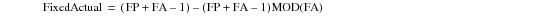The modulo(FA) adjustment aligns the value of FixedActual to the nearest multiple of FA.

15 Calculate the fixed length column overhead (call it B) using the following equation: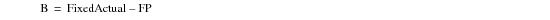16 Set RowSize = FixedActual + FS.

Call this variable CP.

17 Calculate the actual size value (call it CompressActual) for compressible length columns using the following equation: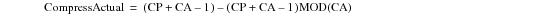The modulo(CA) adjustment aligns the value of CompressActual to the nearest multiple of CA.

18 Calculate the value compressible column overhead (call it C) using the following equation: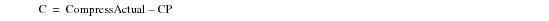19 Set RowSize = CompressActual + CS.

21 Adjust the values of RowSize and 64-Bit_Byte_Alignment_Bit_Overhead if B + C > MAX(FA,CA) using the following pseudocode procedure:

`     IF((B+C) > MAX(FA,CA))`
`      THEN`
`       RowSize = RowSize - CA`
`       Byte_Alignment_Bit_Overhead = Byte_Alignment_Bit_Overhead - CA`
`     END IF`

22 Assign RowSize to VP.

23 Calculate the actual maximum alignment required for variable length columns using the following equation: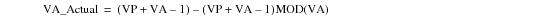The modulo(VA) adjustment aligns the value of VA_Actual to the nearest multiple of VA.

25 Set RowSize = VA_Actual + VS.

26 Calculate the actual value for RowSize using the following equation: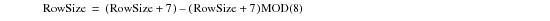The modulo(8) adjustment rounds the value for RowSize upward to the nearest multiple of 8.

27 Calculate the actual value for 64‑Bit_Byte_Alignment_Bit_Overhead using the following equation: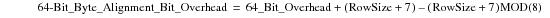The modulo(8) adjustment rounds the value for 64‑Bit_Byte_Alignment_Bit_Overhead upward to the nearest multiple of 8.

28 Calculate the total aligned row format size by adding the values of RowSize and 64‑Bit_Byte_Alignment_Bit_Overhead using the following equation: